## OLS: Ordinary Least Square Method

For those of you who love mathematics and would like to know from how the linear regression formula was derived, in this section of tutorial you will learn a powerful method called Ordinary Least Square (OLS). I assume that you know calculus to perform the OLS method. Knowing this method is important that you may learn to derive many regression formulas by yourselves.

Let us start with notation.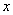is data of independent variable from observation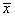is the mean of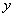is data of dependent variable from observation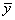is the mean of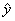is the estimated of, that is represented by the regression model.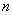is the number of observation data

To perform ordinary least square method, you do the following steps:

1. Set a difference between dependent variable and its estimation: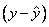2. Square the difference: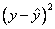3. Take summation for all data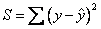4. To get the parameters that make the sum of square difference become minimum, take partial derivative for each parameter and equate it with zero,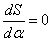For example:

Find for model parameter for model estimation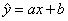using Ordinary Least square!

Answer: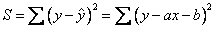The model only has two parameters, that is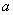and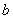.

We take partial derivative of the sum of square difference to the first parameter and equate it to zero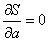. In taking the partial derivative, we assume,andare constant whileis the only variable.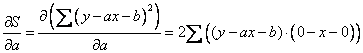Equate it with zero we have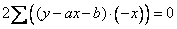Actually, the two parameters,and, are the real constants and they can go out of the summation sign. Constant 2 is surely not equal to zero, thus we can cancel out to simplify.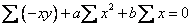We know that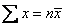, thus we can simplify the last equation into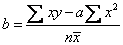(1)

Now, we take partial derivative of the sum of square difference to the second parameter and equate it to zero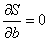. Similar to before, in taking partial derivative, we assume,andare constant, whileis the only variable..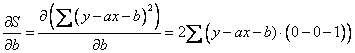Equate it with zero we have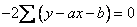Actually, the two parameters,and, are the real constants and they can go out of the summation sign, Constant 2 is surely not equal to zero, thus we can cancel out to simplify.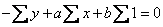We know that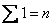and,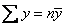, thus we can further simplify the last equation into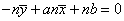or,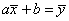(2)

Inputting equation (1) into equation (2), we have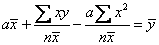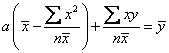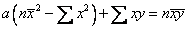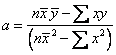Thus, the parameters of regression modelareand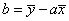Notice that the slopeis actually equivalent to the earlier formula of slope in this tutorial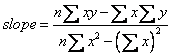by simple algebra.

## Example

Find for model parameter for model estimation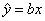using Ordinary Least square!

Answer: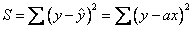The model only has one parameter.

We take derivative and equate it to zero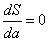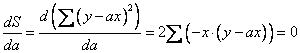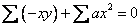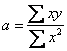Thus, the parameters of regression model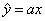is.

You may compare that the slope of the two modelsandare not the same.

Preferable reference for this tutorial is

Teknomo, Kardi (2015) Regression Model using Microsoft Excel. http://people.revoledu.com/kardi/tutorial/Regression/